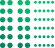# Random Number Generator (RNG)

## Generate a random number or a list of numbers. You can use it as a Random number picker, generate random text numbers, random integers and random decimal numbers

Home

Generator

Random Number GeneratorRefresh
• 9

## Random Number Selection - How to use min and max for different combinations

• ### Random Integer Generator - You can set Integer ranges from -2147483647 to 2147483647

ScenarioMin (inclusive)Max (inclusive) Your Random Number is

10000 99999

### Random Text Number with length 8 to 10

10000000 9999999999

1 2

1 3

1 4

1 5

1 6

1 8

1 9

1 10

1 12

1 20

1 25

1 30

1 50

1 100

500 1000

1 1000

0 9

10 99

100 999

1000 9999

100 999

10000 99999

100000 999999

## Generate Random Numbers with Ease Using a PRNG GeneratorRandom number generators (RNGs) are used to generate sequences of numbers that are unpredictable and unbiased. They are used in a wide variety of applications, including:
• Cryptography: RNGs are used to generate random keys and other cryptographic parameters.
• Gaming: RNGs are used to generate random dice rolls, card draws, and other game events.
• Simulation: RNGs are used to generate random input for computer simulations.
• Statistics: RNGs are used to generate random samples for statistical analysis.
• Security: RNGs are used to generate random passwords and other security credentials.
Important features of a random number generator include:
• Unpredictability: The sequence of numbers generated by the RNG should be unpredictable, even to someone who knows the algorithm used to generate them.
• Unbiasedness: The RNG should generate all possible numbers with equal probability.
• Speed: The RNG should be able to generate numbers quickly enough to meet the needs of the application.
• Efficiency: The RNG should use resources efficiently, such as memory and CPU time.
Some other desirable features of RNGs include:
• Reproducibility: The RNG should be able to generate the same sequence of numbers if given the same initial seed value.
• Portability: The RNG should be able to be implemented on a variety of platforms.
• Ease of use: The RNG should be easy to use and integrate into applications.
There are two main types of random number generators: pseudo-random number generators (PRNGs) and true random number generators (TRNGs). PRNGs use a deterministic algorithm to generate a sequence of numbers that appears to be random. TRNGs use a physical process, such as atmospheric noise or radioactive decay, to generate random numbers.
• PRNGs are more common than TRNGs because they are easier to implement and more efficient. However, TRNGs are more secure because they are more difficult to predict.
• PRNGs work by using a seed value to initialize the algorithm. The seed value is a number that is used to start the algorithm and determine the sequence of numbers that it generates. Once the algorithm is initialized, it generates a sequence of numbers using a mathematical formula.
• The specific features that are important for a random number generator to be useful will vary depending on the application. For example, a random number generator used in cryptography must be very unpredictable and secure, while a random number generator used in gaming does not need to be as secure.
• Overall, random number generators are a versatile tool that can be used in a wide variety of applications. The important features of a random number generator will vary depending on the application, but unpredictability, unbiasedness, speed, and efficiency are all important factors to consider.

Note: The above content has inputs from Bard, the AI model from Google.

## Frequently Asked Questions on RNG Generator